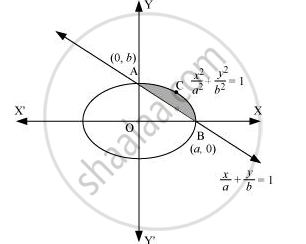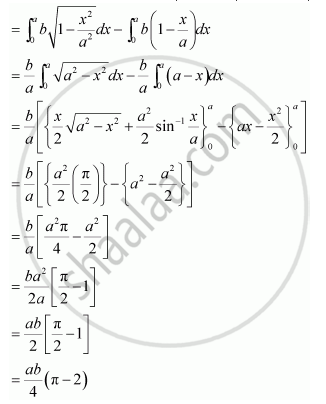Share
Notifications

View all notifications
Books Shortlist
Your shortlist is empty

# Find the Area of the Smaller Region Bounded by the Ellipse X^2/A^2 + Y^2/B^2 = 1 And the Line X/A + Y/B = 1 - CBSE (Commerce) Class 12 - Mathematics

Login
Create free account

Forgot password?

#### Question

Find the area of the smaller region bounded by the ellipse x^2/a^2 + y^2/b^2 = 1 and the line x/a + y/b =   1

#### Solution

The area of the smaller region bounded by the ellipse, x^2/a^2 + y^2/b^2 = 1, and the line, x/a + y/b =   1, is represented by the shaded region BCAB as∴ Area BCAB = Area (OBCAO) – Area (OBAO)Is there an error in this question or solution?

#### APPEARS IN

NCERT Solution for Mathematics Textbook for Class 12 (2018 to Current)
Chapter 8: Application of Integrals
Q: 9 | Page no. 375
Solution Find the Area of the Smaller Region Bounded by the Ellipse X^2/A^2 + Y^2/B^2 = 1 And the Line X/A + Y/B = 1 Concept: Area Under Simple Curves.
S